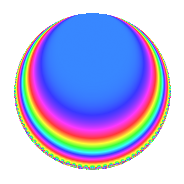# Properties

 Label 4032.2.b.eLevel 4032 Weight 2 Character orbit 4032.b Analytic conductor 32.196 Analytic rank 0 Dimension 2 CM disc. -7 Inner twists 4

# Related objects

## Newspace parameters

 Level: $$N$$ = $$4032 = 2^{6} \cdot 3^{2} \cdot 7$$ Weight: $$k$$ = $$2$$ Character orbit: $$[\chi]$$ = 4032.b (of order $$2$$ and degree $$1$$)

## Newform invariants

 Self dual: No Analytic conductor: $$32.195682095$$ Analytic rank: $$0$$ Dimension: $$2$$ Coefficient field: $$\Q(\sqrt{-7})$$ Coefficient ring: $$\Z[a_1, \ldots, a_{7}]$$ Coefficient ring index: $$2$$ Sato-Tate group: $\mathrm{U}(1)[D_{2}]$

## $q$-expansion

Coefficients of the $$q$$-expansion are expressed in terms of $$\beta = \sqrt{-7}$$. We also show the integral $$q$$-expansion of the trace form.

 $$f(q)$$ $$=$$ $$q$$ $$-\beta q^{7}$$ $$+O(q^{10})$$ $$q$$ $$-\beta q^{7}$$ $$+ 2 \beta q^{11}$$ $$+ 2 \beta q^{23}$$ $$+ 5 q^{25}$$ $$-2 q^{29}$$ $$-6 q^{37}$$ $$-2 \beta q^{43}$$ $$-7 q^{49}$$ $$-10 q^{53}$$ $$+ 6 \beta q^{67}$$ $$+ 2 \beta q^{71}$$ $$+ 14 q^{77}$$ $$+ 6 \beta q^{79}$$ $$+O(q^{100})$$ $$\operatorname{Tr}(f)(q)$$ $$=$$ $$2q$$ $$\mathstrut +\mathstrut O(q^{10})$$ $$2q$$ $$\mathstrut +\mathstrut 10q^{25}$$ $$\mathstrut -\mathstrut 4q^{29}$$ $$\mathstrut -\mathstrut 12q^{37}$$ $$\mathstrut -\mathstrut 14q^{49}$$ $$\mathstrut -\mathstrut 20q^{53}$$ $$\mathstrut +\mathstrut 28q^{77}$$ $$\mathstrut +\mathstrut O(q^{100})$$

## Character Values

We give the values of $$\chi$$ on generators for $$\left(\mathbb{Z}/4032\mathbb{Z}\right)^\times$$.

 $$n$$ $$127$$ $$577$$ $$1793$$ $$3781$$ $$\chi(n)$$ $$-1$$ $$-1$$ $$1$$ $$1$$

## Embeddings

For each embedding $$\iota_m$$ of the coefficient field, the values $$\iota_m(a_n)$$ are shown below.

For more information on an embedded modular form you can click on its label.

Label $$\iota_m(\nu)$$ $$a_{2}$$ $$a_{3}$$ $$a_{4}$$ $$a_{5}$$ $$a_{6}$$ $$a_{7}$$ $$a_{8}$$ $$a_{9}$$ $$a_{10}$$
3583.1
 0.5 + 1.32288i 0.5 − 1.32288i
0 0 0 0 0 2.64575i 0 0 0
3583.2 0 0 0 0 0 2.64575i 0 0 0
 $$n$$: e.g. 2-40 or 990-1000 Significant digits: Format: Complex embeddings Normalized embeddings Satake parameters Satake angles

## Inner twists

Char. orbit Parity Mult. Self Twist Proved
1.a Even 1 trivial yes
7.b Odd 1 CM by $$\Q(\sqrt{-7})$$ yes
4.b Odd 1 yes
28.d Even 1 yes

## Hecke kernels

This newform can be constructed as the intersection of the kernels of the following linear operators acting on $$S_{2}^{\mathrm{new}}(4032, [\chi])$$:

 $$T_{5}$$ $$T_{11}^{2}$$ $$\mathstrut +\mathstrut 28$$ $$T_{19}$$